# Homology of compact non-orientable surfaces

This article describes the value (and the process used to compute it) of some homotopy invariant(s) for a topological space or family of topological spaces. The invariant is homology and the topological space/family is compact non-orientable surface
Get more specific information about compact non-orientable surface | Get more computations of homology

## Statement

Suppose$k$ is a positive integer. We denote by$P_n$ (not standard notation, should try to find something) the connected sum of the real projective plane with itself$n$ times, i.e., the connected sum of$n$ copies of the real projective plane.

### Unreduced version over the integers

We have:$H_k(P_n;\mathbb{Z}) = \lbrace\begin{array}{rl} \mathbb{Z}, & k = 0 \\ \mathbb{Z}^{n-1} \oplus \mathbb{Z}/2\mathbb{Z}, & k = 1\\ 0, & k \ge 2 \\\end{array}$

### Reduced version over the integers

We have:$\tilde{H}_k(P_n;\mathbb{Z}) = \lbrace\begin{array}{rl} 0, & k = 0 \\ \mathbb{Z}^{n-1} \oplus \mathbb{Z}/2\mathbb{Z}, & k = 1\\ 0, & k \ge 2 \\\end{array}$

### Unreduced version over a module

If we consider the homology with coefficients in a module$M$ over a ring$R$ where 2 is invertible, then we have:$H_k(P_n;M) = \lbrace\begin{array}{rl} M, & k = 0 \\ M^{n-1}, & k = 1\\0, & k \ge 2 \\\end{array}$

## Homology groups with integer coefficients in tabular form

We illustrate how the homology groups work for small values of$n$. Note that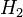$H_2$ is zero and all higher$H_p$ are zero.$n$$P_n$$H_0(P_n)$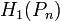$H_1(P_n)$$H_2(P_n)$
1 real projective plane$\mathbb{Z}$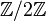$\mathbb{Z}/2\mathbb{Z}$ 0
2 Klein bottle$\mathbb{Z}$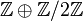$\mathbb{Z} \oplus \mathbb{Z}/2\mathbb{Z}$ 0
3 Dyck's surface$\mathbb{Z}$$\mathbb{Z} \oplus \mathbb{Z} \oplus \mathbb{Z}/2\mathbb{Z}$ 0

## Related invariants

These are all invariants that can be computed in terms of the homology groups.

Invariant General description Description of value for connected sum of$n$ copies of real projective plane Comment
Betti numbers The$k^{th}$ Betti number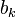$b_k$ is the rank of the torsion-free part of the$k^{th}$ homology group.$b_0 = 1$,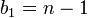$b_1 = n - 1$, all higher$b_k$ are zero
Poincare polynomial Generating polynomial for Betti numbers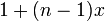$1 + (n - 1)x$
Euler characteristic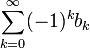$\sum_{k=0}^\infty (-1)^k b_k$$2 - n$ In particular, this means that the Euler characteristic is negative for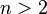$n > 2$. Note that if the Euler characteristic of a compact surface is odd and at most$1$, then the surface must be non-orientable and its homeomorphism type can be computed (using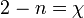$2 - n = \chi$. If the Euler characteristic is even and at most$0$, then there is a unique possibility for a compact orientable surface and a unique possibility for a compact non-orientable surface. For an Euler characteristic of$2$, there is a unique compact orientable surface and no compact non-orientable surface. For an Euler characteristic bigger than$2$, there is no (connected) compact surface possible.